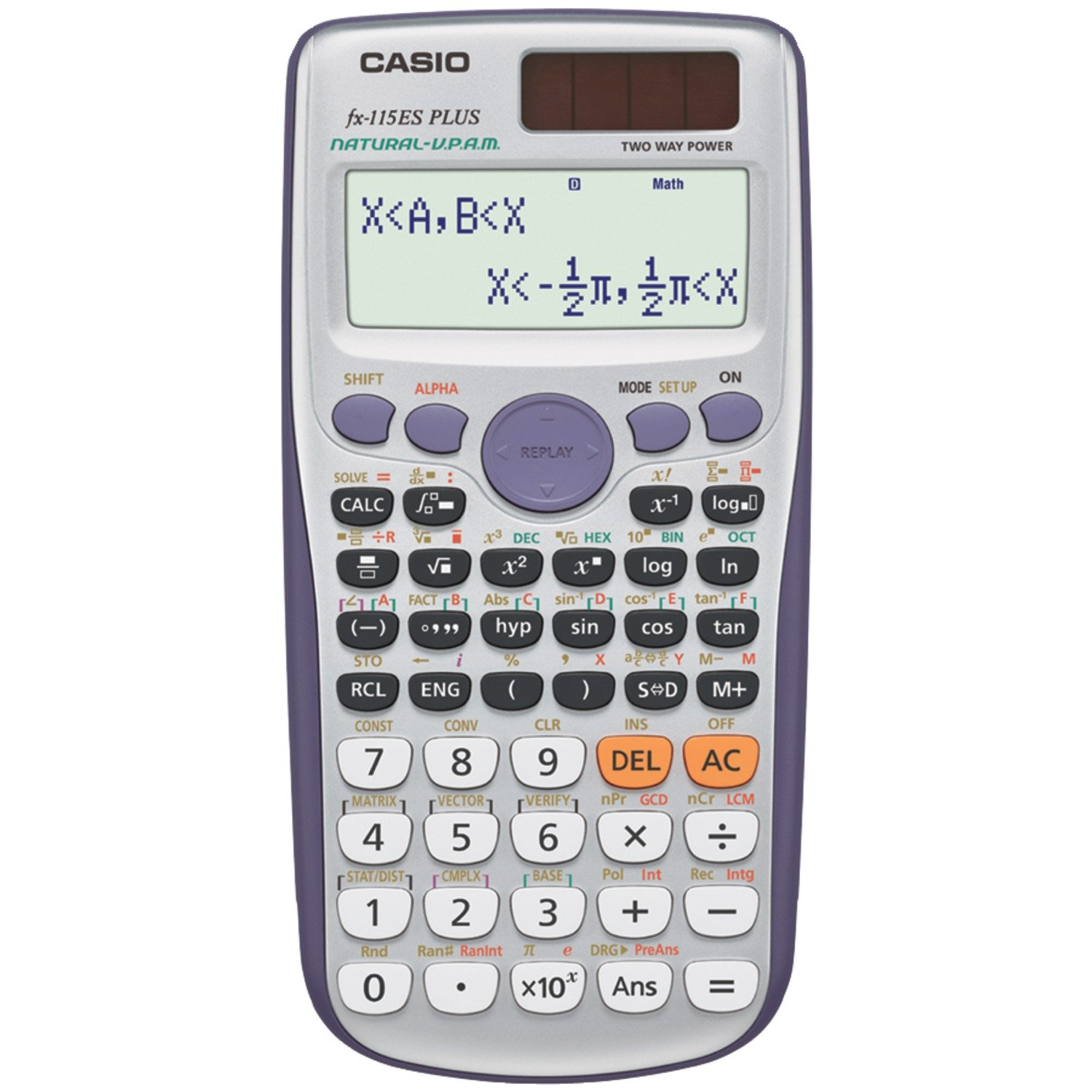Casio
Casio fx-115ES PLUS Engineering/Scientific Calculatorویژگی‌ها
• features "Natural textbook display shows expressions and results exactly as they appear in the textbook" "Over 280 functions including fractions statistics complex number calculations base arithmetic linear regression standard deviation computer science and polar-rectangular conversions" "Multi-replay function allows you to backtrack step-by-step through calculations where you can edit expressions and recalculate answers"وزن
227 g
آلبوم تصاویر